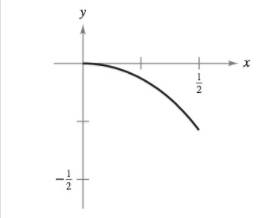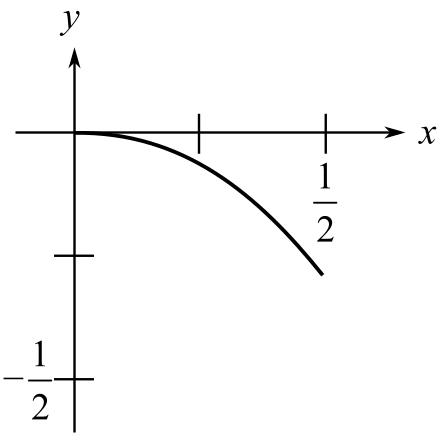Chapter 8, Problem 6PS

Chapter
Section
Textbook Problem

Arc Length Find the arc length of the graph of the function y = ln ( 1 − x 2 ) on the interval 0 ≤ x ≤ 1 2 (see figure).To determine

To calculate: The arc length of the provided graph of function y=ln(1x2) on [0,12].Explanation

Given:

The function y=ln(1x2) on interval [0,12] with graph

Formula Used:

The arc length is determined using the formula

Arc=ab1+(y)2dx

The Derivative formula is

ddxxn=nxn1

ddxlogx=1x

Calculation:

The function is y=ln(1x2).

Differentiating with respect to x, we get

y=ddx(ln(1x2))=1(1x2)(2x)=2x(1x2)

Squaring and adding 1 on both sides, we get

1+(y)2=1+(2x1x2)2=1+4x2(1x2)2=12x2+x4+4x2(1x2)2=1+2x2+x4(1x2)2

Simplify further to get

1+(y)2=1+2x2+(x2)2(1x2)2=(1+x2)2(1x2)2=(1+x21x2)2

Now, find the arc length as shown below

Still sussing out bartleby?

Check out a sample textbook solution.

See a sample solution

The Solution to Your Study Problems

Bartleby provides explanations to thousands of textbook problems written by our experts, many with advanced degrees!

Get Started

Evaluate tanxdx.

Calculus: Early Transcendentals

Find a formula for the inverse of the function. 26. y=1ex1+ex

Single Variable Calculus: Early Transcendentals, Volume I

Find the mean, median, and mode for the following scores; 8, 7, 5, 7, 0, 10, 2, 4, 11, 7, 8, 7

Essentials of Statistics for The Behavioral Sciences (MindTap Course List)

Add and reduce to lowest terms. 4514+1659+13

Contemporary Mathematics for Business & Consumers

∫ sin 3x cos 6x dx =

Study Guide for Stewart's Single Variable Calculus: Early Transcendentals, 8th

For and , a × b =

Study Guide for Stewart's Multivariable Calculus, 8th

What is the goal of a single-case experimental research design?

Research Methods for the Behavioral Sciences (MindTap Course List)